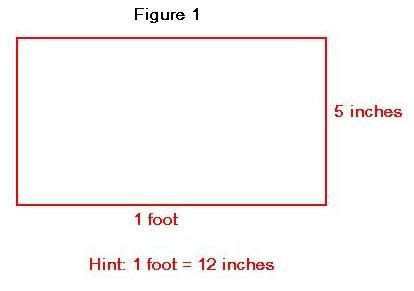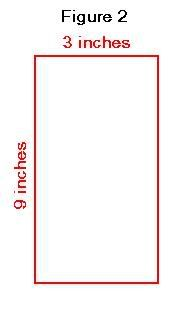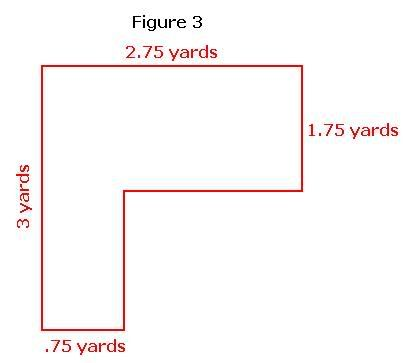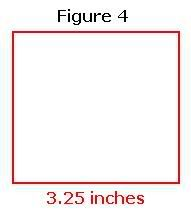## Apr 9, 2009

### Area and Perimeter - Square and Rectangle

1) Find the perimeter and area of a rectangle with width 6 feet and length 14 feet.

Solution:

Length = 14 ft

Width = 6 ft

Perimeter of a rectangle = 2(l + w) = 2(14+6) = 2(20) = 40 ft.

Area of a rectangle = length * width = 14 * 6 = 84 square feet.

2) Find the area and perimeter of a rectangle with length 42 and width 12.

Solution:

Length = 42

Width = 12

Perimeter of a rectangle = 2(l + w) = 2(42+12) = 2(54) = 108 units.

Area of a rectangle = length * width = 42 * 12 = 504 square units.

--------------------------------------------------------------------------------------------------------

Practice questions:

i) Find the perimeter and area of a rectangle with length 12 yards and width 6 yards.

ii) Find the perimeter and area of a rectangle whose length is 5 yards and width is 6 feet. ( Hint: 3 feet = 1 yard )

iii) Find the area and perimeter of a given rectangle ( see figure1)iv) Length and width of a rectangle is 11 inches and 5 inches respectively. Find its area and perimeter.

v) In a rectangle, length = 12.5 cm and width = 7.5 cm. Find its area and perimeter.

vi) Dimensions of a rectangle are given in figure 2. Find the area and perimeter of a rectangle.vii) If the length and width of a rectangle is 39 inches and 2 feet respectively, find its area and perimeter.(Hint: 1 foot = 12 inches)

viii) Find the area and perimeter for a given figure 3.ix) Area of a rectangle is 25 sq. cm. What is the total area of 5 such rectangles?

x) The length and width of a rectangle is 3 inches and 5 inches respectively. Find the perimeter of a rectangle formed by joining the width of such 3 rectangles.

------------------------------------------------------------------------------------------------

3) Find the perimeter and area of a square with side 11 meters.

Solution:

Side =11 m

Perimeter of a square = 4 * side = 4 * 11 = 44 m

Area of a square = side * side = 9 * 9 = 81 sq. m

4) Find the area and perimeter of a square with side 5.5 cm.

Solution:

Side =5.5 cm

Perimeter of a square = 4 * side = 4 * 5.5 = 22 cm

Area of a square = side * side = 5.5 * 5.5 = 30.25 sq. cm

-------------------------------------------------------------------------------------------------

Practice questions:

i) Find the perimeter and area of a square with side 12 feet.

ii) Find the area and perimeter of a given square ( see figure 4)iii) Find the perimeter and area of a square with side 8.2 cm.

iv) If the side of a square measures 4 yards, find its perimeter and area.

v) Find the area and perimeter of a given figure 5.vi) Find the area of a square of length 12 yards. Also find its perimeter.

vii) Area of a square is 2 sq. ft. What is the area of a figure formed by 20 such squares?

viii) Length of a square is 8 cm. How many such squares can be arranged to form a rectangle of length 24 cm and width 16cm.

ix) Length and width of a rectangle is 4.5 inches and 2 inches respectively. If a square is perfectly attached to the width of the rectangle, what is the total area of the new rectangle formed?

x) A rectangle with length 10 yards and width 4 yards are cut into squares. What is the maximum possible area of a square?